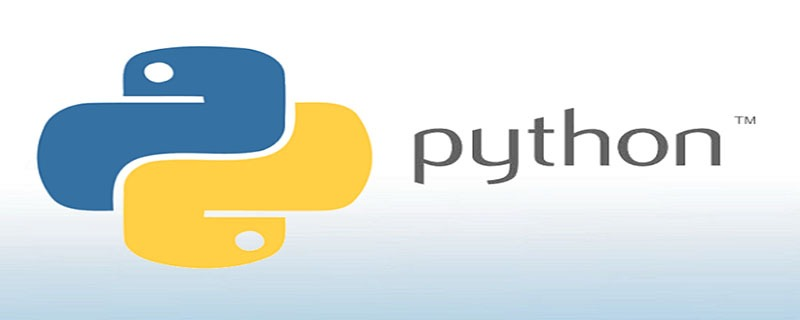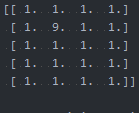## python如何定义矩阵

1,911次阅读```>>> import numpy as np
>>> y=np.empty([2,2],dtype=int)   #表示定义一个2*2的矩阵，该矩阵为整型```

```import numpy as np
x,y = map(int,input().split())
a = np.ones((x+1,y+1))``````import numpy as np
x,y = map(int,input().split())
a = np.ones((x+1,y+1))
a = 9
print(a)```